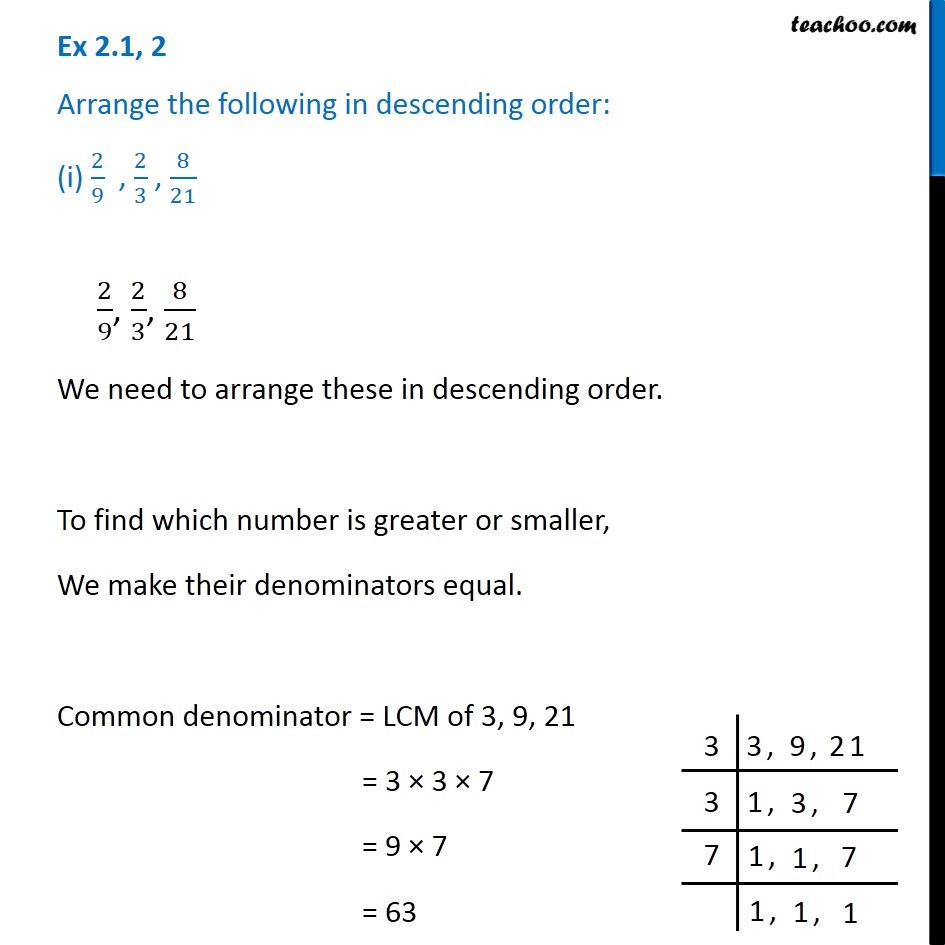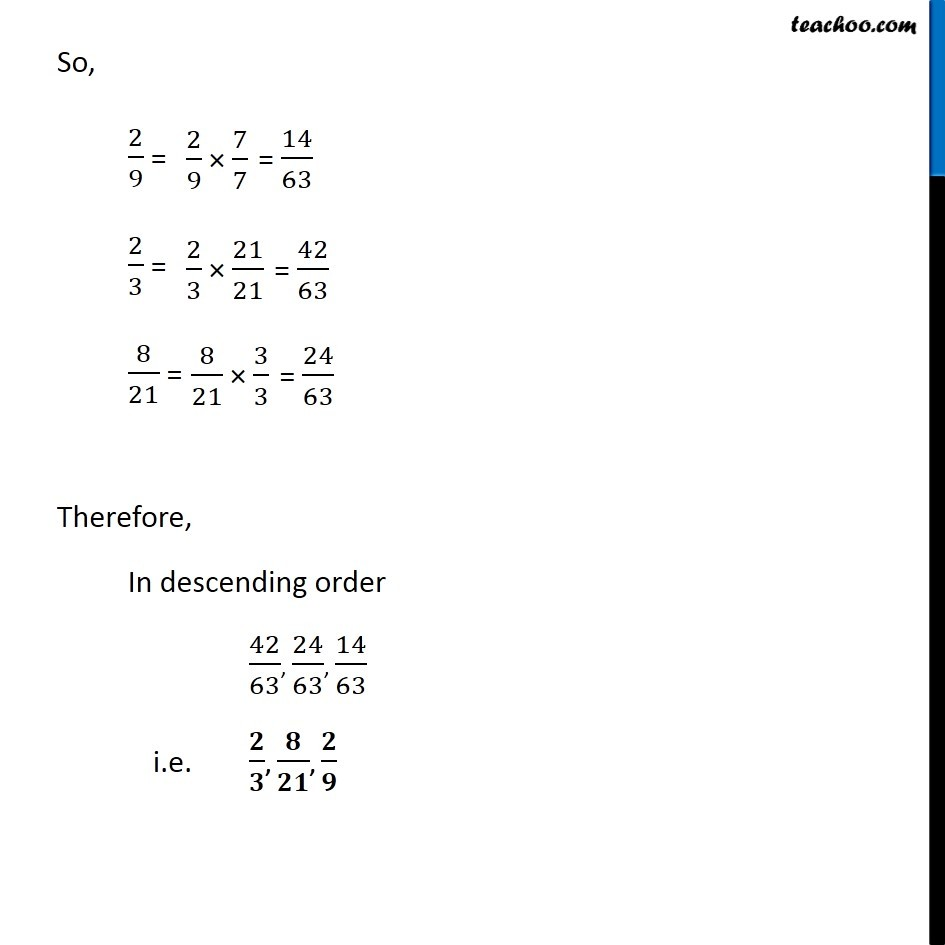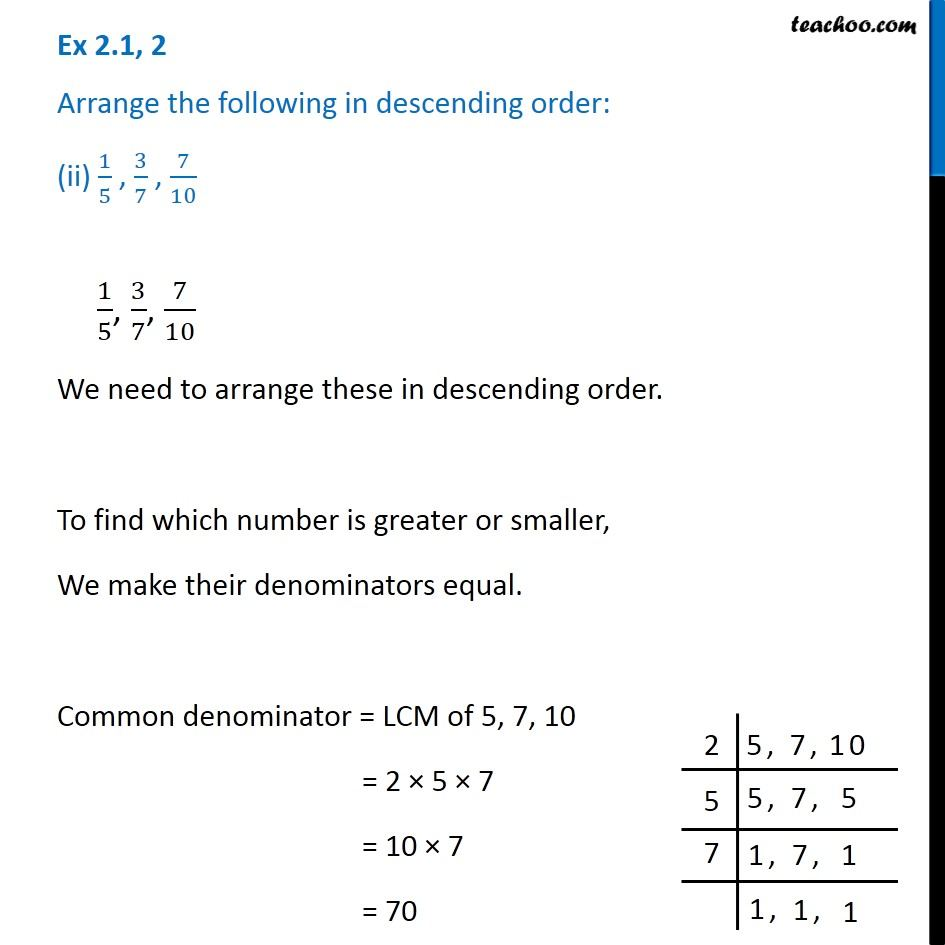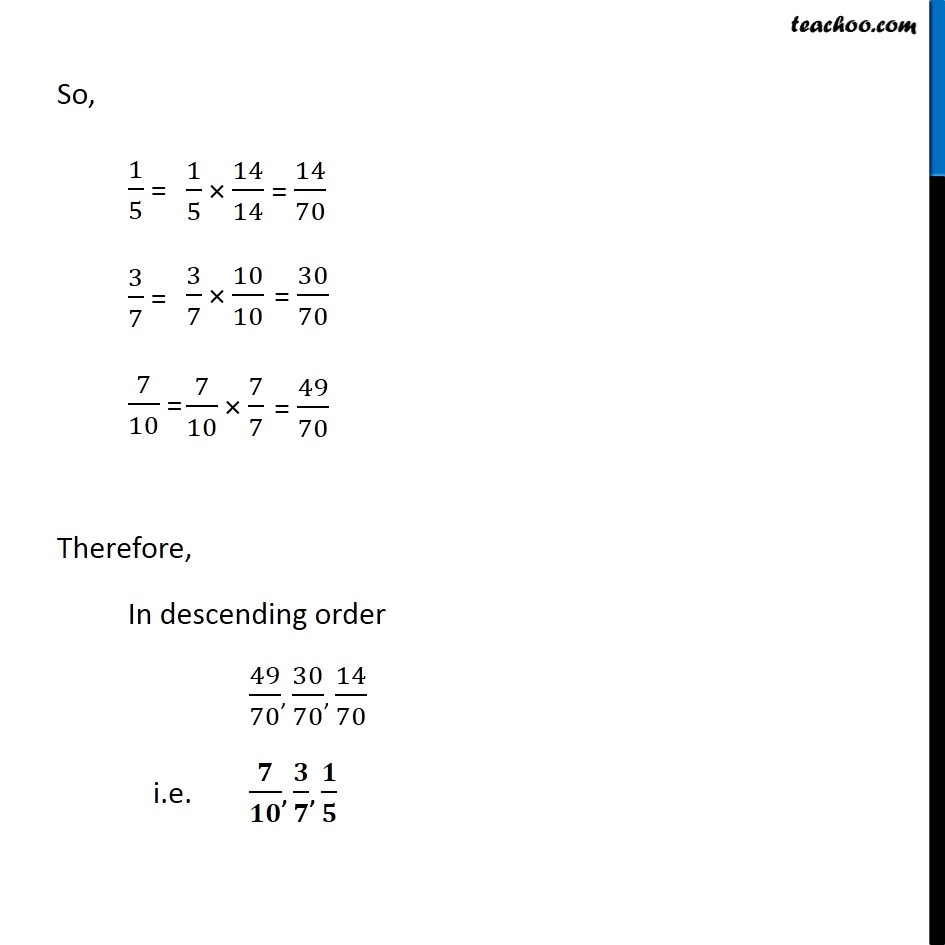1. Chapter 2 Class 7 Fractions and Decimals
2. Concept wise
3. Greater than, less than in fractions

Transcript

Ex 2.1, 2 Arrange the following in descending order: (i) 2/9 , 2/3 , 8/21 2/9, 2/3, 8/21 We need to arrange these in descending order. To find which number is greater or smaller, We make their denominators equal. Common denominator = LCM of 3, 9, 21 = 3 3 7 = 9 7 = 63 So, 2/9 = 2/3 = 8/21 = Therefore, In descending order 42/63, 24/63, 14/63 i.e. / , / , / Ex 2.1, 2 Arrange the following in descending order: (ii) 1/5 , 3/7 , 7/10 1/5, 3/7, 7/10 We need to arrange these in descending order. To find which number is greater or smaller, We make their denominators equal. Common denominator = LCM of 5, 7, 10 = 2 5 7 = 10 7 = 70 So, 1/5 = 3/7 = 7/10 = Therefore, In descending order 49/70, 30/70, 14/70 i.e. / , / , /

Greater than, less than in fractions